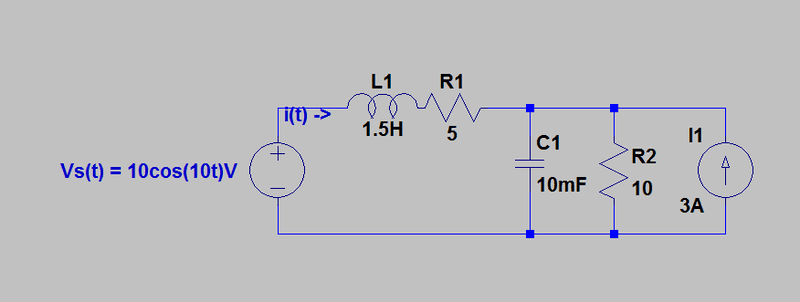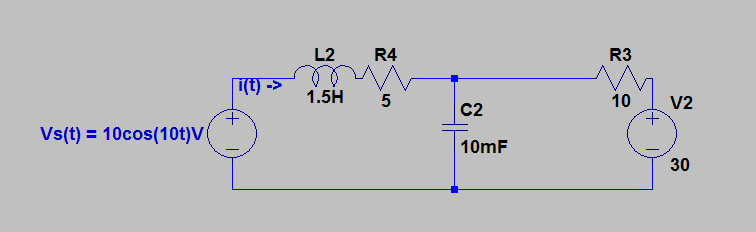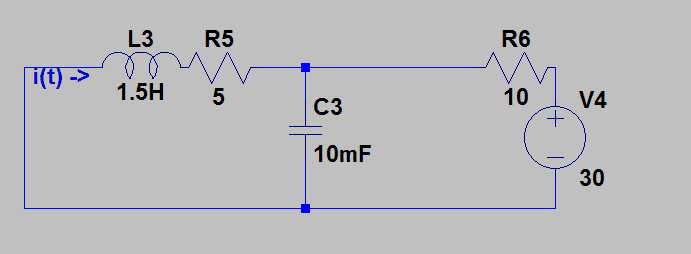# Superposition with AC voltage source and DC current source

## Homework Statement

Using the superposition principle, determine the current i(t.2. Homework Equations

Zc = 1/jwC
Zl = jwL
V = I*R
I = V/Z

## The Attempt at a Solution

First, I converted inductor/capacitor to impedance:
L = 1.5H -> jwL = j * 10 * 1.5 = 15j
C = 10mf = 1/(jwC) = 1/(j*10*10*10^-3) = -0.1j

Then, I transformed the 3A DC current source and 10 ohm resistor into a DC voltage source:I then shorted the 30V DC source to find i(t) with only Vs(t) = 10cos(10t)V
After shorting the 30V DC source, C2 and R3 are in parallel. Their impedance is:

(0.1j * 10)/(10-0.1j) = -j/(10-0.1j)
After rationalizing: (0.1 - j)/100.01

Now that, L2 and R4 are in series, total impedance:
15j + 5 + (0.1 - j)/100.01 = (500.15-1499.15j)/100.01 = 15.8 < -71.55°.
I = V/Z = (10<0°)/(15.88<-71.55° ) = 10/15.88<71.55°A

Now I short the AC source:The DC source had a frequency of 0 rad/s, so the impedance of C3 is infinity (1/0) and L3 is 0 (j*0*L).
The inductor is shorted and the capacitor is opened. There is a voltage source of 30V and a combined resistance of 15ohms. V=IR -> I = 30/15 = 2A<0°
[(500.15/100.01 + 2) + (1499.15j/100.01)]
Is 16.54<64.96° A the correct answer?

Last edited:

gneill
Mentor

•eehelp150
Is it -10j instead of -0.1j?

gneill
Mentor
Is it -10j instead of -0.1j?
It is indeed!

•eehelp150
It is indeed!
My new current for part 1 is 10/14.14 <-45°
Is the final answer (2.5 + 0.5j) A?

gneill
Mentor
That looks good!

•eehelp150
gneill
Mentor
That looks good!
Oops, hang on. I think your signs may be incorrect. The diagram defines the current direction as flowing out of the AC source. So the DC contribution to that must be negative.

•eehelp150
gneill
Mentor
My new current for part 1 is 10/14.14 <-45°
Is the final answer (2.5 + 0.5j) A?
Also, if the AC current angle is -45°, its imaginary part should be negative...

•eehelp150
Also, if the AC current angle is -45°, its imaginary part should be negative...
It was negative on my paper, I don't know why I typed positive.
(-1.5 - 0.5j)A, correct?

gneill
Mentor
Yes, that looks good.

•eehelp150
Yes, that looks good.
Thank you so much for your help!

Yes, that looks good.
the final answer is -1.5 - 0.5j amps
If w = 10, would the answer in time domain be:
$$\sqrt{2.5} * cos(10t + 18.435°)A$$
?

gneill
Mentor
The DC and AC components must be kept separate. There will be a constant DC current with an AC component superimposed on it, or if you like, the AC signal will be offset by a DC bias.

The DC and AC components must be kept separate. There will be a constant DC current with an AC component superimposed on it, or if you like, the AC signal will be offset by a DC bias.
How would I write it out? I need the answer in the time domain.

gneill
Mentor
How would I write it out? I need the answer in the time domain.
A DC current is just a fixed value in the time domain; a number. So your result should have the form:

##I(t) = I_{dc} + I_{ac}##

where ##I_{ac}## is your cosine expression (the part that varies with time).

A DC current is just a fixed value in the time domain; a number. So your result should have the form:

##I(t) = I_{dc} + I_{ac}##

where ##I_{ac}## is your cosine expression (the part that varies with time).
So:

I(t) = sqrt(2)/2 * cos(10t - 45°) - 2

gneill
Mentor
Yes. That looks better!